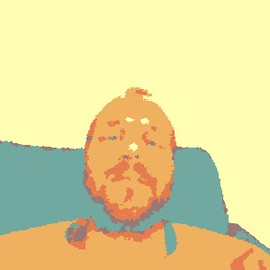# Generative Art Gifs

## Setup

This script is inspired mostly by packages and a blog post of Thomas Pedersen. To understand the code, I recommend to read the blog post first. And you should know R. Otherwise, maybe scroll directly to the gifs 😄.

``````library(ambient)
library(tidyverse)``````

Furthermore, the packages {paletteer}, {animation}, {here} and {tweenr} are needed.

Let’s define the gif width / height:

``````anim_height = 300
anim_width = 400``````

Define grid:

``````grid <- long_grid(x = seq(0, 1, length.out = anim_width),
y = seq(0, 1, length.out = anim_height))``````

``````grid_simplex <- grid %>%
mutate(
perturb = gen_simplex(x, y, frequency = 5) / 3
)``````

## Where are we?

GIF based on plot method with increasing wave frequencies

The function `ambient::gen_waves()` can generate wave patterns from this 2-dimensional simplex grid.

Create list of grids based on an increasing sequence of frequencies (we take the same simplex noise `perturb` defined before):

``````freqs <- seq(1, 3, length.out = 50)

grid_list <-
freqs %>%
map(~ mutate(grid_simplex,
noise = gen_waves(x + perturb,
y + perturb,
frequency = .x)
)
)``````

This list of grids is plotted subsequently to produce a gif (the reverse list is first added to produce a smooth gif):

``````animation::saveGIF(
{
c(grid_list, rev(grid_list)) %>% map( ~ plot(.x, noise))
},
interval = 1 / 10,
movie.name = here::here("ambient_anim_plot.gif"),
ani.height = anim_height,
ani.width = anim_width
)``````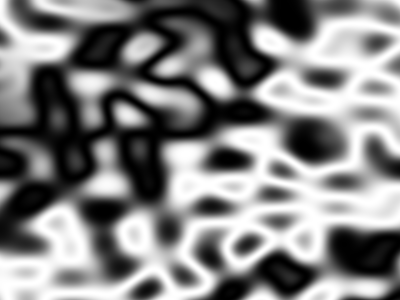Increasing wave frequency gif based on ggplot with custom color palette

The same can be done using ggplot instead of plot. Here, we can use the custom color palettes provided by {paletteer} (this should also be possible with plot, but I had problems using the as.raster() plot method).

``````ggplot_ambient <- function(long_grid, pal) {
p <-
ggplot(long_grid %>% as_tibble(), aes(x = x, y = y, fill = noise)) +
geom_raster() +
theme_void() +
theme(legend.position = "none")  +
paletteer::scale_fill_paletteer_c(pal)
# it's important to print() the output of the function for saveGIF to work:
print(p)
}
# A single plot could now be produced via:
# grid_list[] %>% as_tibble() %>% ggplot_ambient("oompaBase::blueyellow")
# (using the palette "oompaBase::blueyellow")

# save thumbnail for blog post:
# grid_list[] %>%
#   ggplot_ambient("oompaBase::jetColors") %>%
#   ggsave(here::here("content", "post", "2020-04-04-generative-art-gifs", "featured.png"),
#          .,
#          height = anim_width/300,
#          width = anim_height/300,
#          dpi = 300)``````

Now we can produce the gif with ggplot in the same way as before with plot:

``````animation::saveGIF(
{
c(grid_list, rev(grid_list)) %>% map(~ggplot_ambient(.x, "scico::berlin"))
},
interval = 1 / 25,
movie.name = here::here("ambient_ggplot.gif"),
ani.height = anim_height,
ani.width = anim_width
)``````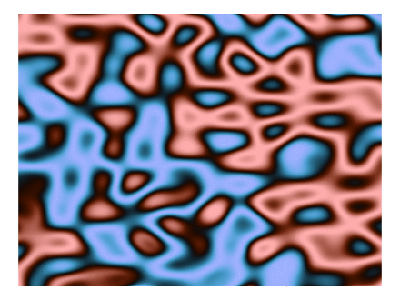## Lava lamps from outer space

tweening between different noise images

The next gif will be produced by tweening between different simplex noise grids.

Instead of switching the wave frequency it is also possible to tween between images of different simplex noises. First we create 2 more simplex grids (a new seed is used every time we call gen_simplex()):

``````grid_simplex2 <- grid %>%
mutate(
perturb = gen_simplex(x, y, frequency = 5) / 3
)
grid_simplex3 <- grid %>%
mutate(
perturb = gen_simplex(x, y, frequency = 5) / 3
)``````

The next function will tween between two different grids grid_simplex & grid_simplex2, by creating a grid list as before. However, this time we’ll keep the frequency constant, but create nframes = 50 transitions states:

``````gen_tween_grid <- function(grid_simplex,
grid_simplex2,
nframes = 50) {
grid_simplex_seq <-
tweenr::tween_states(list(grid_simplex, grid_simplex2),
tweenlength = 3,
statelength = 0,
# ease = 'back-in-out',
ease = 'linear',
nframes = nframes) %>%
group_split(.frame)

grid_wave2 <-
map(grid_simplex_seq,
~mutate(.x,
noise = gen_waves(x + perturb,
y + perturb,
frequency = 1)
)
)
}``````

With this function we can tween between our 3 simplex grids (Taking 3 looks more interesting than back-and-forthing between two as before, because in the latter case there would be regions which hardly change color in the resulting gif):

``````animation::saveGIF(
{
c(
gen_tween_grid(grid_simplex, grid_simplex2),
gen_tween_grid(grid_simplex2, grid_simplex3),
gen_tween_grid(grid_simplex3, grid_simplex)
) %>%
map(~ggplot_ambient(.x, "oompaBase::jetColors"))
},
interval = 1 / 20,
movie.name = here::here("ambient_tween.gif"),
ani.height = anim_height,
ani.width = anim_width
)``````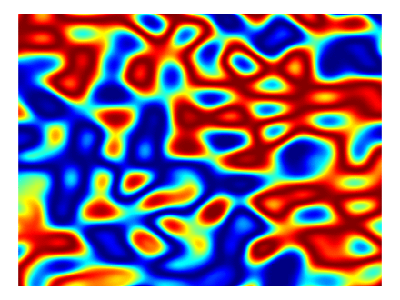## Traversing the rainbow nebula

GIF by using the third dimension of ambient::long_grid

After having done that I realized that instead of tweening one can also create smooth transitions by using a third dimension when creating the simplex noise:

``````grid3d <- long_grid(
seq(0, 1, length.out = anim_width),
seq(0, 1, length.out = anim_height),
# In the 3rd dimension I take a smaller range, because otherwise the gif
# would move a bit fast:
seq(0, 0.2, length.out = 50)
) %>%
mutate(perturb = gen_simplex(x, y, z, frequency = 5) / 3)``````

We just need to produce a list by splitting this data.frame on the 3rd coordinate (which will be our time coordinate in the gif):

``````grid3d_list <-
grid3d %>%
mutate(noise = gen_waves(x + perturb,
y + perturb,
z + perturb)) %>%
group_split(z, keep = FALSE)``````
``````## Warning: The `keep` argument of `group_split()` is deprecated as of dplyr 1.0.0.
## This warning is displayed once every 8 hours.
## Call `lifecycle::last_warnings()` to see where this warning was generated.``````

Now we can create our gif as above:

``````animation::saveGIF(
{
c(grid3d_list, rev(grid3d_list)) %>%
map(~ggplot_ambient(.x, "oompaBase::jetColors"))
},
interval = 1 / 10,
movie.name = here::here("ambient3d.gif"),
ani.height = anim_height,
ani.width = anim_width
)``````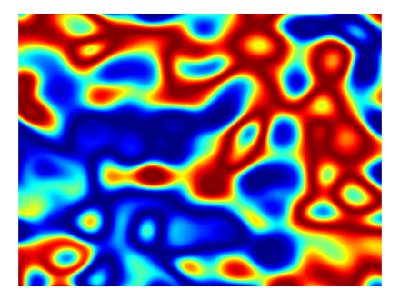## Cold smoke

use gganimate and another color palette

Of course, I already tried gganimate when I started to use ggplot to produce the gifs. But somehow it didn’t work and I didn’t understand the error messages. After having discovered to use the 3rd dimension of ambient::long_grid for the gifs, I thought I should give it another try. Here is how it can be done:

``````anim_height = 300
anim_width = 400
nframes = 50
grid3d <- long_grid(
seq(0, 1, length.out = anim_width),
seq(0, 1, length.out = anim_height),
# In the 3rd dimension I take a smaller range, because otherwise the gif
# would move a bit fast:
seq(0, 0.2, length.out = nframes)
) %>%
mutate(perturb = gen_simplex(x, y, z, frequency = 5) / 3)

anim <-
grid3d %>%
mutate(noise = gen_waves(x + perturb,
y + perturb,
z + perturb)) %>%
ggplot(aes(x = x, y = y, fill = noise)) +
geom_raster() +
# Let's take another palette:
paletteer::scale_fill_paletteer_c("scico::hawaii") +
theme_void() +
theme(legend.position = "none") +
gganimate::transition_states(z, transition_length = 0)

gganimate::anim_save(here::here("gganim.gif"),
anim,
# because we rewind
nframes = 2 * nframes,
fps = 20,
rewind = TRUE,
width = anim_width,
height = anim_height)``````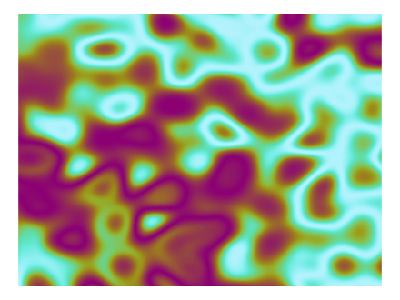Really cool, isn’t it?! If you have questions or comments, I would be really happy if you drop me a note. Thank you for all the hard work of the involved software used! It’s all for free and I really love these packages 🤩.